December 4, 2019

## Meetup Presentations

• 8.32 Neil Shah

## Bayesian Analysis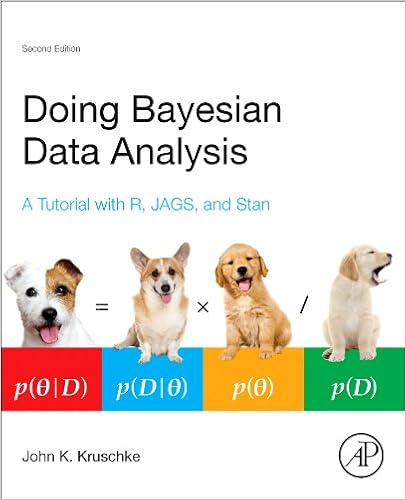Kruschke’s videos are an excelent introduction to Bayesian Analysis https://www.youtube.com/watch?v=YyohWpjl6KU!

Doing Bayesian Data Analysis, Second Edition: A Tutorial with R, JAGS, and Stan

The Theory That Would Not Die: How Bayes’ Rule Cracked the Enigma Code, Hunted Down Russian Submarines, and Emerged Triumphant from Two Centuries of Controversy by Sharon Bertsch McGrayne

Video series by Rasmus Baath Part 1, Part 2, Part 3

## Bayes Theorem

$P\left( A|B \right) =\frac { P\left( B|A \right) P\left( A \right) }{ P\left( B|A \right) P\left( A \right) +P\left( B|{ A }^{  } \right) P\left( { A }^{  } \right) }$

Consider the following data from a cancer test:

• 1% of women have breast cancer (and therefore 99% do not).
• 80% of mammograms detect breast cancer when it is there (and therefore 20% miss it).
• 9.6% of mammograms detect breast cancer when it’s not there (and therefore 90.4% correctly return a negative result).
Cancer (1%) No Cancer (99%)
Test postive 80% 9.6%
Test negative 20% 90.4%

## How accurate is the test?

Now suppose you get a positive test result. What are the chances you have cancer? 80%? 99%? 1%?

• Ok, we got a positive result. It means we’re somewhere in the top row of our table. Let’s not assume anything - it could be a true positive or a false positive.
• The chances of a true positive = chance you have cancer * chance test caught it = 1% * 80% = .008
• The chances of a false positive = chance you don’t have cancer * chance test caught it anyway = 99% * 9.6% = 0.09504
Cancer (1%) No Cancer (99%)
Test postive True +: 1% * 80% False +: 99% * 9.6% 10.304%
Test negative False -: 1% * 20% True -: 99% * 90.4% 89.696%

## How accurate is the test?

$Probability = \frac{desired\quad event}{all\quad possibilities}$

The chance of getting a real, positive result is .008. The chance of getting any type of positive result is the chance of a true positive plus the chance of a false positive (.008 + 0.09504 = .10304).

$P(C | P) = \frac{P(P|C) P(C)}{P(P)} = \frac{.8 * .01}{.008 + 0.095} \approx .078$

So, our chance of cancer is .008/.10304 = 0.0776, or about 7.8%.

## Bayes Formula

It all comes down to the chance of a true positive result divided by the chance of any positive result. We can simplify the equation to:

$P\left( A|B \right) =\frac { P\left( B|A \right) P\left( A \right) }{ P\left( B \right) }$

## How many fish are in the lake?

• Catch them all, count them. Not practical (or even possible)!
• We can sample some fish.

Our strategy:

1. Catch some fish.
2. Mark them.
3. Return the fish to the pond. Let them get mixed up (i.e. wait a while).
4. Catch some more fish.
5. Count how many are marked.

For example, we initially caught 20 fish, marked them, returned them to the pond. We then caught another 20 fish and 5 of them were marked (i.e they were caught the first time).

## Strategy for fitting a model

Step 1: Define Prior Distribution. Draw a lot of random samples from the “prior” probability distribution on the parameters.

n_draw <- 100000
n_fish <- sample(20:250, n_draw, replace = TRUE)
head(n_fish, n=10)
##   153  67  41  82  90 103  54  48  86 167
hist(n_fish, main="Prior Distribution")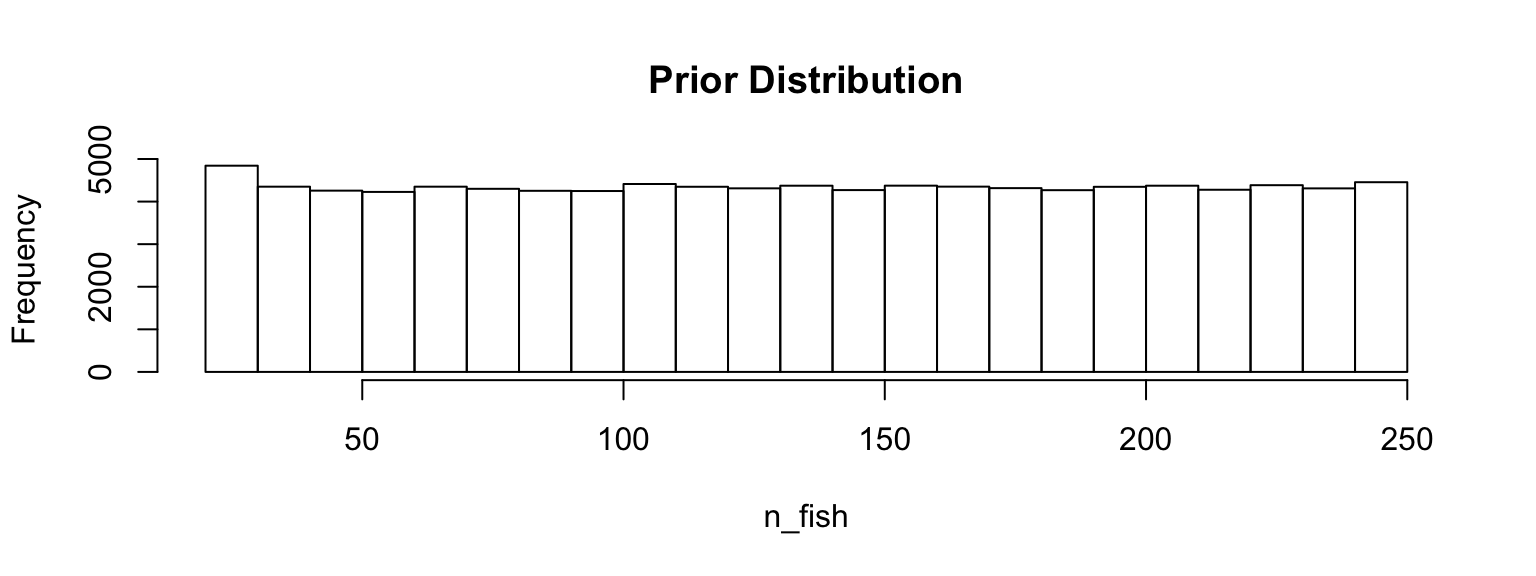## Strategy for fitting a model

Step 2: Plug in each draw into the generative model which generates “fake” data.

pick_fish <- function(n_fish) { # The generative model
fish <- rep(0:1, c(n_fish - 20, 20))
sum(sample(fish, 20))
}
n_marked <- rep(NA, n_draw)
for(i in 1:n_draw) {
n_marked[i] <- pick_fish(n_fish[i])
}
head(n_marked, n=10)
##    3  8 13  5  4  7  8  8  5  2

## Strategy for fitting a model

Step 3: Keep only those parameter values that generated the data that was actually observed (in this case, 5).

post_fish <- n_fish[n_marked == 5]
hist(post_fish, main='Posterior Distribution')
abline(v=median(post_fish), col='red')
abline(v=quantile(post_fish, probs=c(.25, .75)), col='green')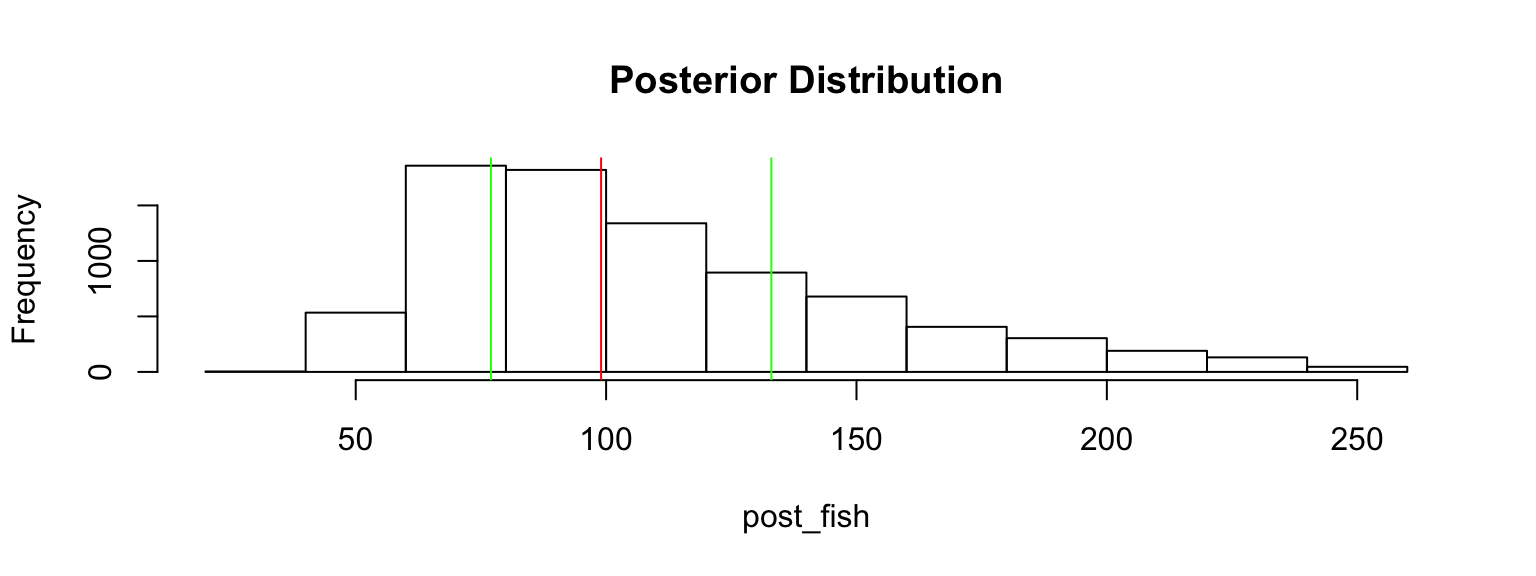## What if we have better prior information?

An “expert” believes there are around 200 fish in the pond. Insteand of a uniform distribution, we can use a binomial distribution to define our “prior” distribution.

n_fish <- rnbinom(n_draw, mu = 200 - 20, size = 4) + 20
hist(n_fish, main='Prior Distribution')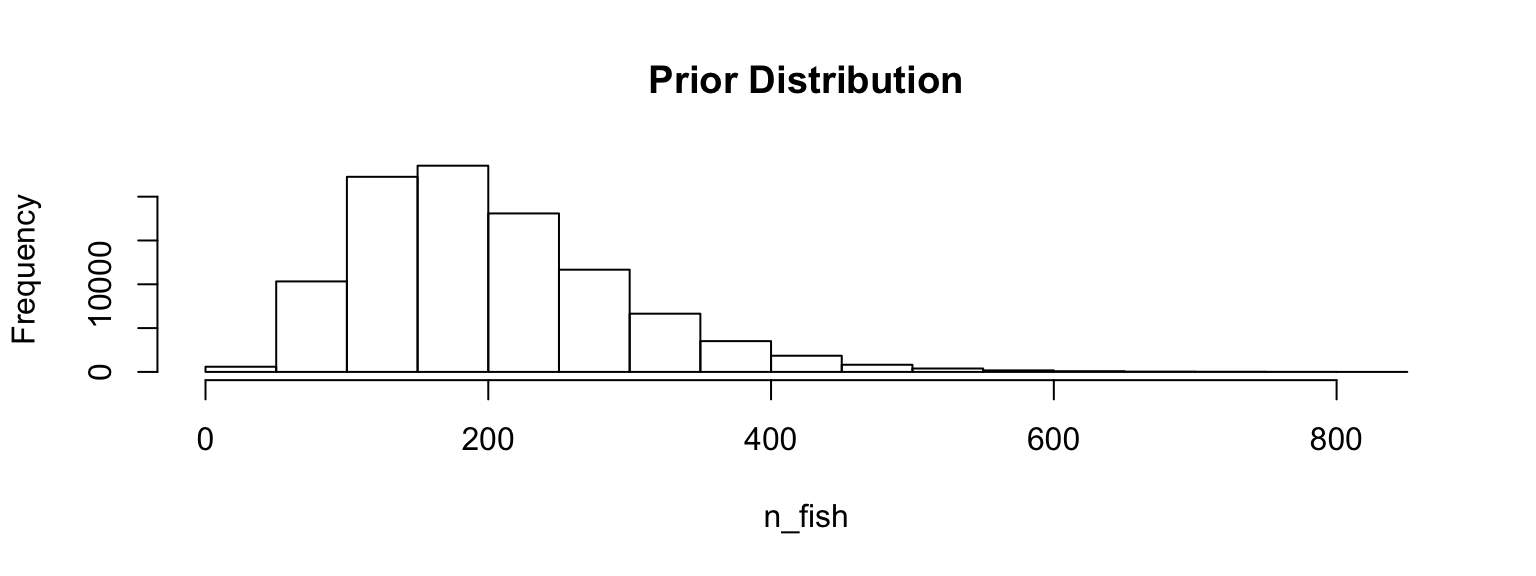## What if we have better prior information?

n_marked <- rep(NA, n_draw)
for(i in 1:n_draw) {
n_marked[i] <- pick_fish(n_fish[i])
}
post_fish <- n_fish[n_marked == 5]
hist(post_fish, main='Posterior Distribution')
abline(v=median(post_fish), col='red')
abline(v=quantile(post_fish, probs=c(.25, .75)), col='green')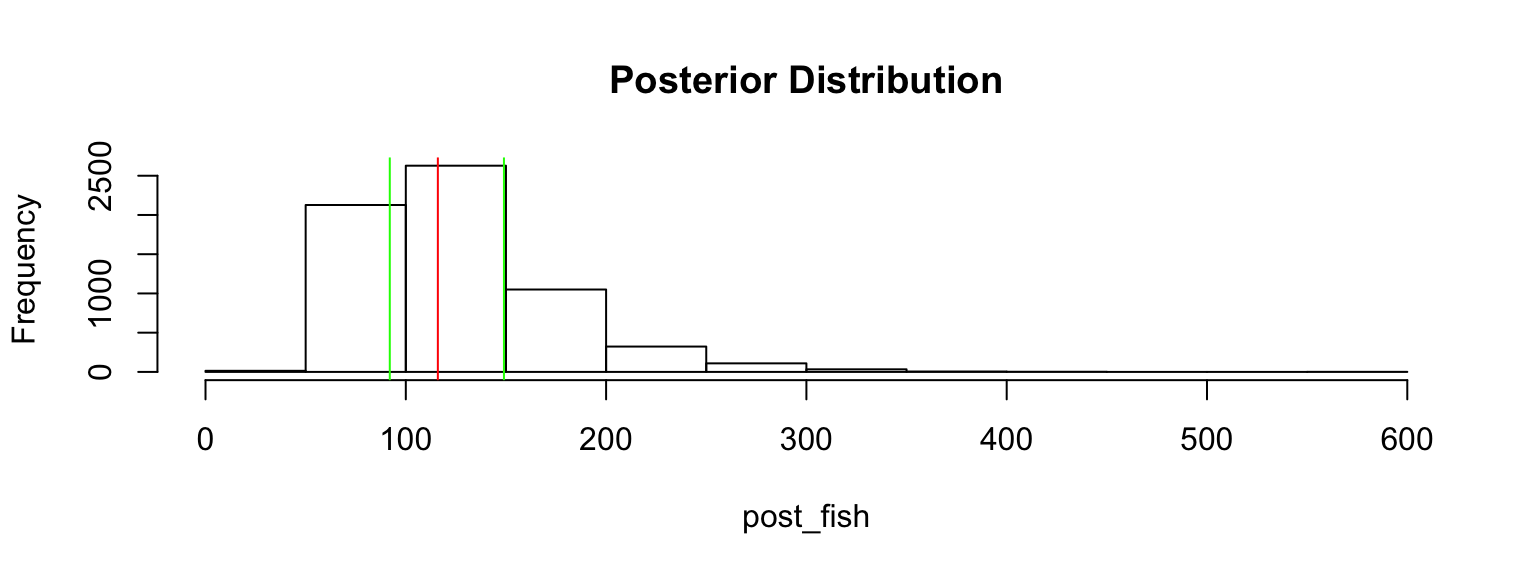## Bayes Billiards Balls

Consider a pool table of length one. An 8-ball is thrown such that the likelihood of its stopping point is uniform across the entire table (i.e. the table is perfectly level). The location of the 8-ball is recorded, but not known to the observer. Subsequent balls are thrown one at a time and all that is reported is whether the ball stopped to the left or right of the 8-ball. Given only this information, what is the position of the 8-ball? How does the estimate change as more balls are thrown and recorded?

shiny_demo('BayesBilliards', package='DATA606')